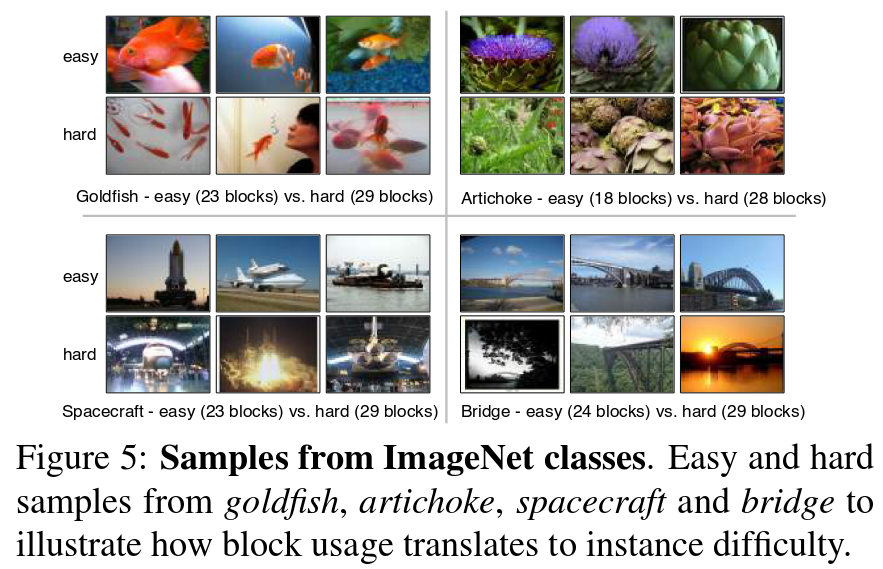The authors propose a method that can predict, by looking at an image with a small CNN, which residual blocks to keep in a large Resnet at inference. According to the hypothesis that ResNets are ensembles of independent paths, blocks can be dropped while keeping useful signal going up to the classification head. The method is trained using RL; more specifically Policy Gradient.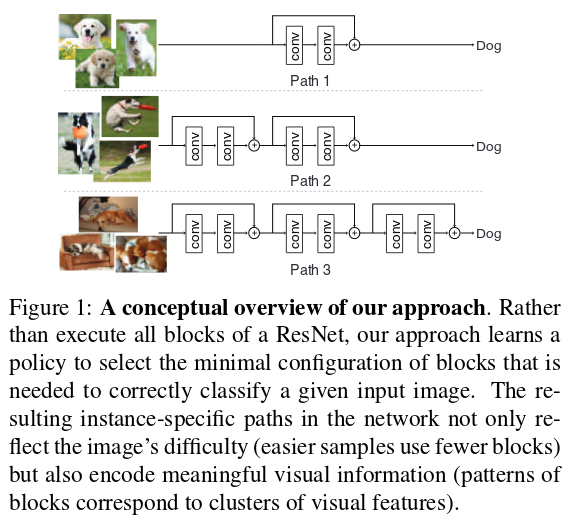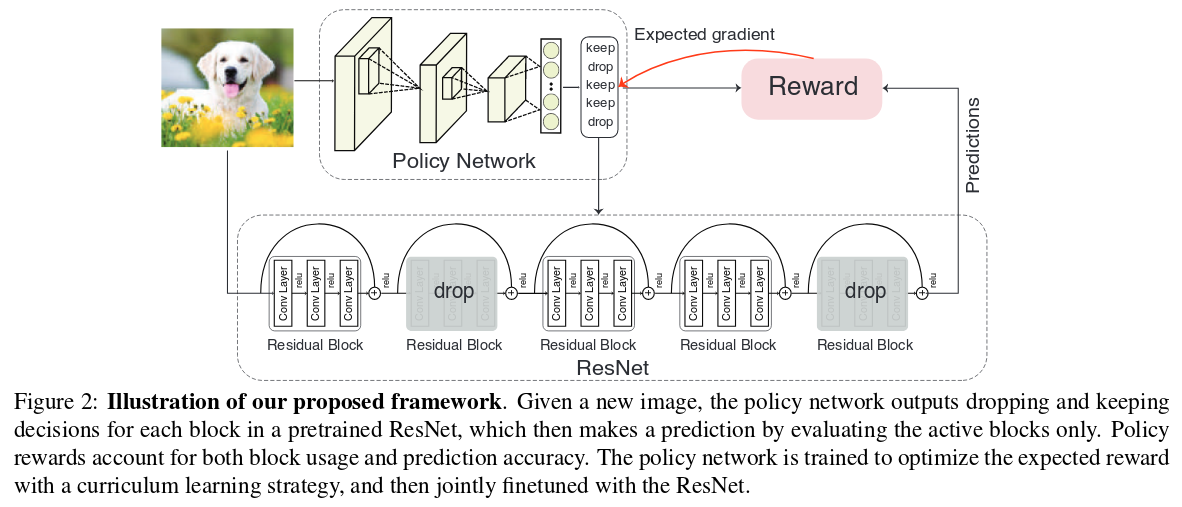# Reward Function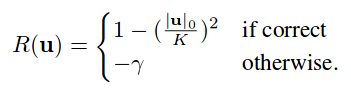$$\mathbf{u}$$ is the policy vector describing which blocks to keep; $$K$$ is the total number of blocks; and $$\gamma$$ is the accuracy/speed trade-off parameter.

# Curriculum Learning

Since there is no ground truth for the policy vectors, it is very hard to learn a policy for the whole ResNet from scratch. Curriculum Learning helps: they keep the first $$N$$ residual blocks and predict a policy vector only for the remaining blocks, decreasing $$N$$ as the policy network gets better. Eventually, $$N = 0$$ and the policy predicts the structure of the whole ResNet.

# Quantitative Results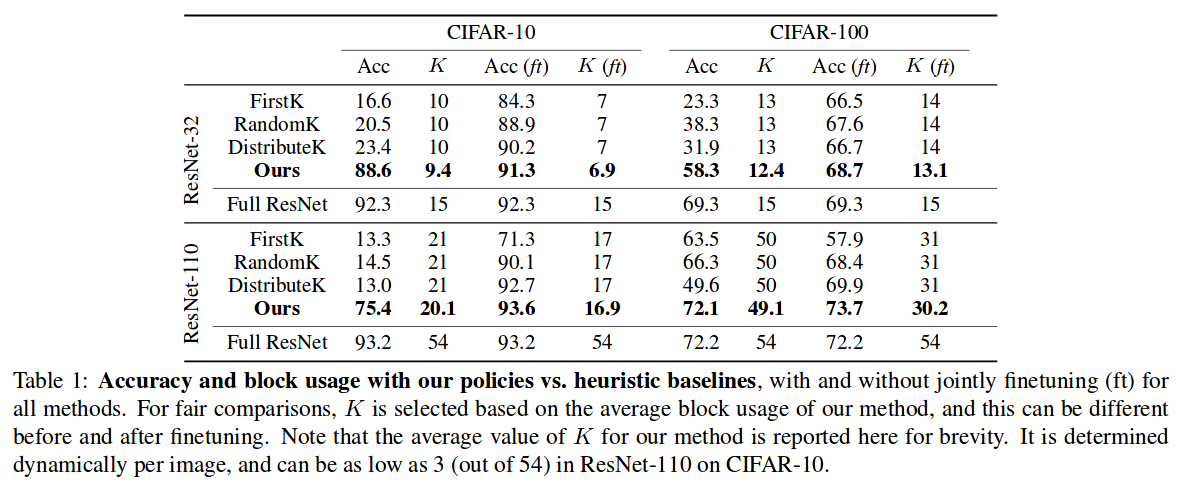With ResNet-101, an average speedup of 20% is obtained, which is an order of magnitude less than the $$2 \times$$ speedup typically yielded by pruning methods. However, BlockDrop is orthogonal to pruning; both approaches could be implemented at once.

# Qualitative Results

In a class-wise manner, the authors have selected 3 frequently occuring policy vectors, and show some of the corresponding instances: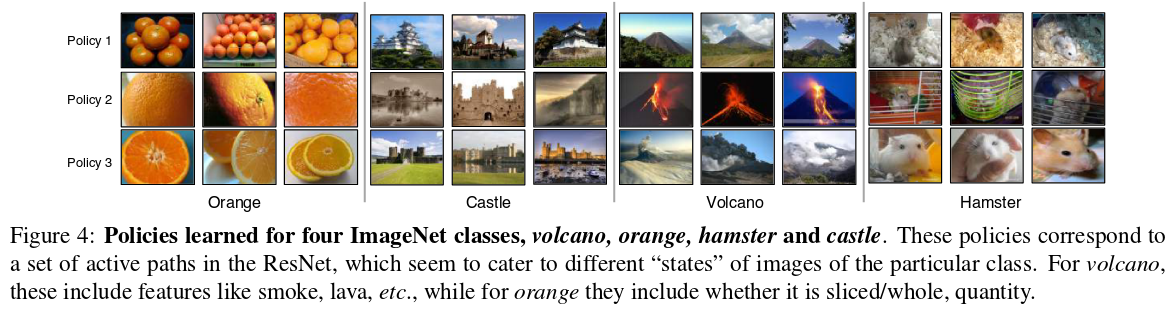The authors also discuss the link between image difficulty and the number of blocks used: